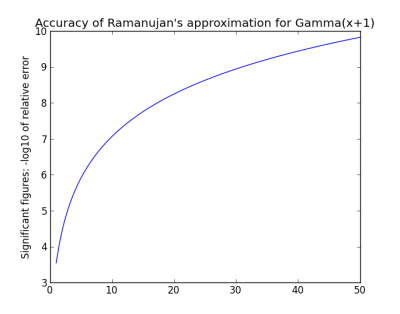# Ramanujan’s factorial approximation

Ramanujan came up with an approximation for factorial that resembles Stirling’s famous approximation but is much more accurate.As with Stirling’s approximation, the relative error in Ramanujan’s approximation decreases as n gets larger. Typically these approximations are not useful for small values of n. For n = 5, Stirling’s approximation gives 118.02 while the exact value is 120. But Ramanujan’s approximation gives 120.00015.

Here’s an implementation of the approximation in Python.

    def ramanujan(x):
fact = sqrt(pi)*(x/e)**x
fact *= (((8*x + 4)*x + 1)*x + 1/30.)**(1./6.)
return fact


For non-integer values of x, the function returns an approximation for Γ(x+1), an extension of factorial to real values. Here’s a plot of the accuracy of Ramanujan’s approximation.For x = 50, Ramanujan’s approximation is good to nearly 10 significant figures, whereas Stirling’s approximation is good to about 7.

Here’s a little trickier implementation.

    def ramanujan2(x):
fact = sqrt(pi)*(x/e)**x
fact *= (((8*x + 4)*x + 1)*x + 1/30.)**(1./6.)
if isinstance(x, int):
fact = int(fact)
return fact


This code gives the same value as before if x is not an integer. But it gives exact values of factorial for x = 0, 1, 2, … 10. If x is 11 or greater, the result is not exact, but the relative error is less than 10^-7 and decreases as x increases. Ramanujan’s approximation always errs on the high side, so rounding the result down improves the accuracy and makes it exact for small integer inputs.

The downside of this trick is that now, for example, ramanujan2(5) and ramanujan2(5.0) give different results. In some contexts, the improved accuracy may not be worth the inconsistency.

Related post: A Ramanujan series for calculating pi## 12 thoughts on “Ramanujan’s factorial approximation”

1. You can get rid of the ramanujan2(5) and ramanujan2(5.0) inconsistency by the following method:
 def ramanujan2(x): fact = math.sqrt(math.pi)*(x/math.e)**x fact *= (((8*x + 4)*x + 1)*x + 1/30.)**(1./6.) if (x - int(x) == 0): fact = int(fact) return fact 
so rather than checking it’s type you can compare numerically to it’s integer value.

2. David: Your code eliminates the inconsistency I mentioned. However, there is another issue I didn’t mention in the post.

Your function will have a slight discontinuity near integer values. Let’s call your function ramanujan3. For some very small value of ε, ramanujan3(5 - ε) will return a larger value than ramanujan3(5) even though Γ(x+1) should be an increasing function. While ramanujan2 is less accurate than ramanujan3, it is an increasing function as long as you give it floating point type arguments.

I’m straining at gnats in this example. If you want high accuracy, there are better algorithms. My point, however, is that sometimes the more accurate implementation is not preferable. In some contexts, you might give up a little accuracy for continuity or monotonicity.

3. alfC: Yes, built-in gamma and log gamma functions are more accurate. If you have access to such functions, by all means use them.

But if you were in an environment where you didn’t have access to such functions and you didn’t need high accuracy, you could write your own in a couple lines of code using this approximation.

4. I wonder how on earth Ramanujan came up with such formulae …

5. The idea is to start not with what we call Stirling’s approximation but the full asymptotic series whose first term is what we call Stirling’s approximation. Next, rather than truncating (i.e. lopping off) the rest of series, approximate the rest of the series.

6. Bill Ricker

solution to ramanujan #2/3 dilemma —

Let the caller call int(ramanujan(5)) or with 5.0 too if they want the exactitude and don’t care about gamma continuity (for combinatorics) and let them not if they really want gamma.

However, this is misleading, as the excess exceeds 1 and even 2 as low as ramanujan(11). The answer grows even faster than this phenomenal accuracy.

Better that FACT returns a float for 11 as a warning that it’s not exact !

7. Pseudonym

We don’t know how Ramanujan came up with his approximations, because he violated the high school maths rule by never showing his working.

8. Pseudonym

Oh, incidentally, I use Ramanujan’s approximation all the time. For some reason, my work needs log(n!) more than it needs n!.

9. Another problem: ramanujan(171) returns an ›good‹ approximation (inf), butramanujan2(171) fails: isinstance(x, int) is true, but  fact = int(fact) throws an OverflowError because such large floats can’t be represented as integers.

10. Gerry Jensen

Hello,
using P 2.7.9,
i tried your code using either gmpy2 or math for the term
(x/e)**x,
and either got
“mpz does not support infinity” for gmpy2 or
“overflow 34, result too large” for math, using
fact = math.sqrt(math.pi)*(x/math.e)**x

What library are you using or do i need to go to Python 3?

Also, using this approximation, would you expect to be missing more large primes than smaller ones, considering their distribution?